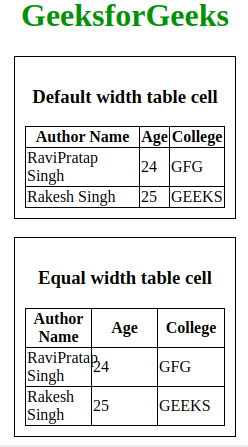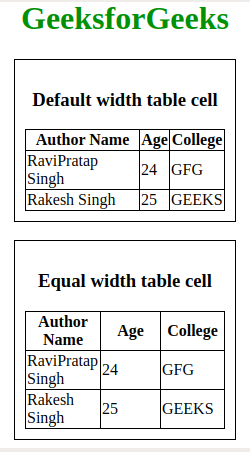# How to create equal width table cell using CSS ?

HTML tables are created using the table tag with the combination of subsequent tags like tr tag to create a new row in the table. The th tag for table headers and td tag is used for defining a cell in the table. However, each cell of the table is by default designed to fit content of any size hence each changes its width according to the information inside it. Hence we learn how to fix the width of a cell to any size or value.

By default the HTML cell size is dynamic. So if the content to be displayed in the cell is large the size of the cell changes according to the content in it. However, there are some scenarios where fixed cell size is required to maintain uniformity and for aesthetic purposes.

There are several ways to make the cell size fixed in HTML tables.

Using CSS table-layout Property: This property should be used for changing the layout features of the table. By default the table-layout value is auto. This means the cell size varies according to the content in it if we changed it to fix that will affect. By changing the value to fixed, the table layout is fixed. Cells in other rows do not affect column widths. If no widths are present on the first row, the column widths. The column widths are divided equally across the table, regardless of content inside the cells.

Below example illustrate the above approach:

• Example:

 ` ` `<``html``> ` ` `  `<``head``> ` `    ``<``title``>table-layout property ` `    ``<``style``> ` `        ``table { ` `            ``border-collapse: collapse; ` `            ``border: 1px solid black; ` `        ``} ` ` `  `        ``th, ` `        ``td { ` `            ``border: 1px solid black; ` `        ``} ` ` `  `        ``table#table1 { ` `            ``table-layout: auto; ` `            ``width: 200px; ` `        ``} ` ` `  `        ``/* Equal width table cell */ ` `        ``table#table2 { ` `            ``table-layout: fixed; ` `            ``width: 200px; ` `        ``} ` ` `  `        ``div { ` `            ``max-width: 200px; ` `            ``padding: 10px; ` `            ``border: 1px solid black; ` `        ``} ` ` `  `        ``h1 { ` `            ``color: green; ` `        ``} ` `    `` ` ` ` ` `  `<``body``> ` `    ``<``center``> ` `        ``<``h1``>GeeksforGeeks ` `        ``<``div``> ` `            ``<``h3``>Default width table cell ` `            ``<``table` `id``=``"table1"``> ` `                ``<``tr``> ` `                    ``<``th``>Author Name ` `                    ``<``th``>Age ` `                    ``<``th``>College ` `                `` ` `                ``<``tr``> ` `                    ``<``td``>RaviPratap Singh ` `                    ``<``td``>24 ` `                    ``<``td``>GFG ` `                `` ` `                ``<``tr``> ` `                    ``<``td``>Rakesh Singh ` `                    ``<``td``>25 ` `                    ``<``td``>GEEKS ` `                `` ` `            `` ` `        ``<``br``> ` `        ``<``div``> ` `            ``<``h3``>Equal width table cell ` `            ``<``table` `id``=``"table2"``> ` `                ``<``tr``> ` `                    ``<``th``>Author Name ` `                    ``<``th``>Age ` `                    ``<``th``>College ` `                `` ` `                ``<``tr``> ` `                    ``<``td``>RaviPratap Singh ` `                    ``<``td``>24 ` `                    ``<``td``>GFG ` `                `` ` `                ``<``tr``> ` `                    ``<``td``>Rakesh Singh ` `                    ``<``td``>25 ` `                    ``<``td``>GEEKS ` `                `` ` `            `` ` `        `` ` `    `` ` ` ` ` `  ` `

• Output:Using CSS width property: By using this property, we can define each cell’s width value. If we set the value in the equal percentage unit then the width of the each cell will be equal, every time window size doesn’t matter.

• Example:

 ` ` `<``html``> ` ` `  `<``head``> ` `    ``<``title``>table-layout property ` `    ``<``style``> ` `        ``table { ` `            ``border-collapse: collapse; ` `            ``border: 1px solid black; ` `        ``} ` ` `  `        ``th, ` `        ``td { ` `            ``border: 1px solid black; ` ` `  `        ``} ` ` `  `        ``table#table2 td { ` `            ``width: 33%; ` `        ``} ` ` `  `        ``div { ` `            ``max-width: 200px; ` `            ``padding: 10px; ` `            ``border: 1px solid black; ` `        ``} ` ` `  `        ``h1 { ` `            ``color: green; ` `        ``} ` `    `` ` ` ` ` `  `<``body``> ` `    ``<``center``> ` `        ``<``h1``>GeeksforGeeks ` `        ``<``div``> ` `            ``<``h3``>Default width table cell ` `            ``<``table` `id``=``"table1"``> ` `                ``<``tr``> ` `                    ``<``th``>Author Name ` `                    ``<``th``>Age ` `                    ``<``th``>College ` `                `` ` `                ``<``tr``> ` `                    ``<``td``>RaviPratap Singh ` `                    ``<``td``>24 ` `                    ``<``td``>GFG ` `                `` ` `                ``<``tr``> ` `                    ``<``td``>Rakesh Singh ` `                    ``<``td``>25 ` `                    ``<``td``>GEEKS ` `                `` ` `            `` ` `        ``<``br``> ` `        ``<``div``> ` `            ``<``h3``>Equal width table cell ` `            ``<``table` `id``=``"table2"``> ` `                ``<``tr``> ` `                    ``<``th``>Author Name ` `                    ``<``th``>Age ` `                    ``<``th``>College ` `                `` ` `                ``<``tr``> ` `                    ``<``td``>RaviPratap Singh ` `                    ``<``td``>24 ` `                    ``<``td``>GFG ` `                `` ` `                ``<``tr``> ` `                    ``<``td``>Rakesh Singh ` `                    ``<``td``>25 ` `                    ``<``td``>GEEKS ` `                `` ` `            `` ` `        `` ` `    `` ` ` ` ` `  ` `

• Output:My Personal Notes arrow_drop_upCheck out this Author's contributed articles.

If you like GeeksforGeeks and would like to contribute, you can also write an article using contribute.geeksforgeeks.org or mail your article to contribute@geeksforgeeks.org. See your article appearing on the GeeksforGeeks main page and help other Geeks.

Please Improve this article if you find anything incorrect by clicking on the "Improve Article" button below.

Practice Tags :

Be the First to upvote.

Please write to us at contribute@geeksforgeeks.org to report any issue with the above content.